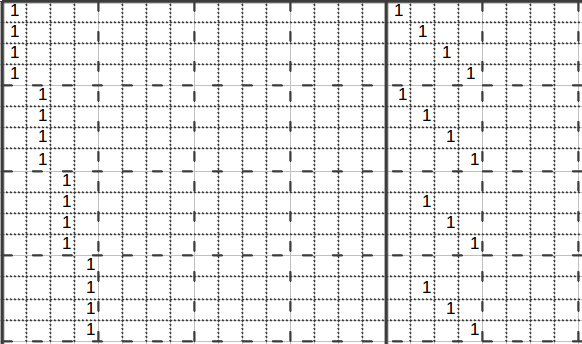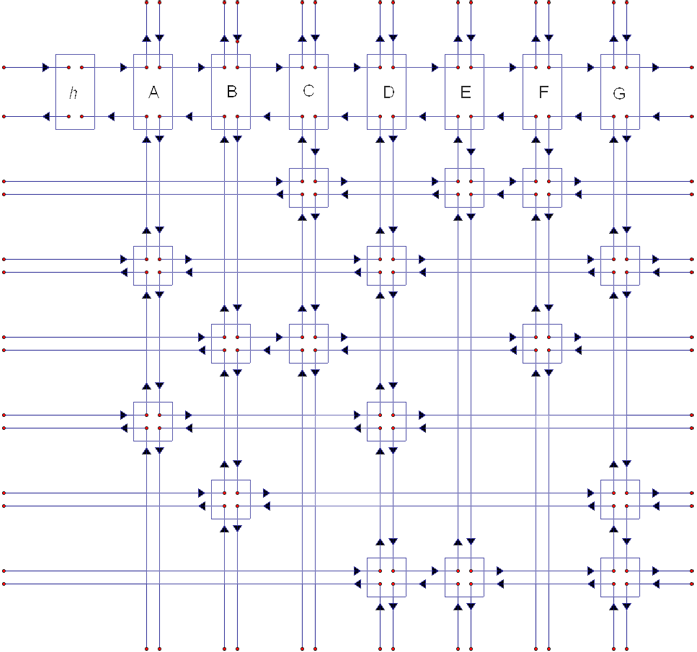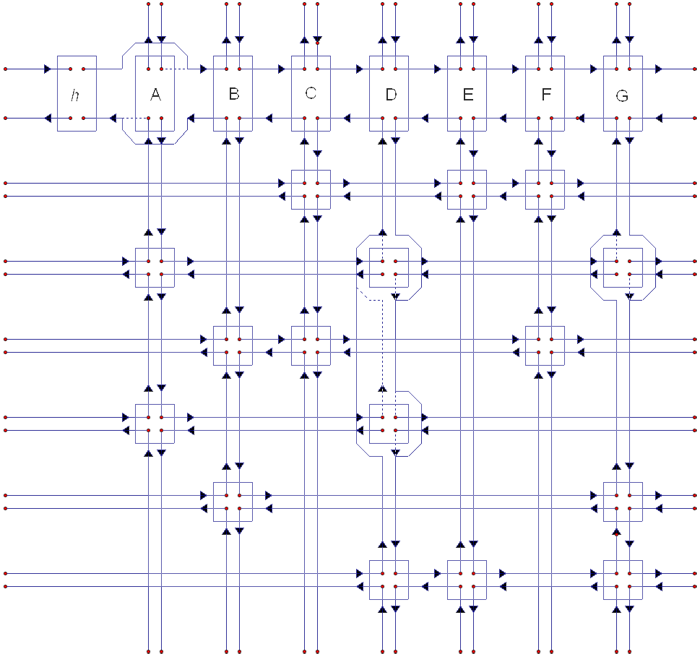# Solving Sudoku Fast

The famous Japanese puzzle has been around since the 19th century. However it wasn’t until the late 90’s that computer program was written to generate puzzles quickly, and even later in 2006 that a fast technique was developed to solve arbitrary sized Sudoku puzzles. The technique reduces Sudoku to a different problem, and utilizes an efficient data-structure to search for a solution. Let’s take a look!

## Solving Sudoku

If we are to solve Sudoku using a bruteforce method, our algorithm would have to try each available number across all empty cells. Such an algorithm would have a runtime complexity of O(N^(N²)), where n is size of the Sudoku puzzle. For a 9x9 Sudoku puzzle (N = 9), the algorithm would perform 2*10⁷⁷ operations to find a solution. That would not be practical. In practice the runtime would vary according to the difficulty of the puzzle itself and the number of options for each empty cell. For example, a 17-clue puzzle with diagonal symmetry is one of the hardest to solve due to the large number of candidates and branches.

## Reduction to Exact Cover

Let us consider the following problem: Given a binary matrix of N rows and M columns, find a subset of the rows where each column sums to 1. So a valid solution will have a single 1 in each column. This is known as the exact cover problem, which is NP-complete. That means any problem in NP can be reduced to the exact cover problem, which is what makes NP-complete problems so interesting. Graph-coloring, 2D Knapsack, Tiling, and Sudoku are all reducible to an exact cover problem.

Let us represent Sudoku in terms of an exact cover problem. We will transform the 4 constraints of a Sudoku puzzle into a binary matrix such that a solution to the exact cover matrix will have a one to one mapping to a solution to the Sudoku puzzle. The constraints for any Sudoku puzzle are:

• For each row, a number can appear only once.
• For each column, a number can appear only once.
• For each region (small square), a number can appear only once.
• Each cell can only have one number.

In our binary matrix, each column will represent a constraint for a Sudoku cell and each row will represent a possible assignment for a Sudoku cell to a candidate number. For 9x9 Sudoku, the binary matrix will have 4 x 9² columns and 9³ rows. The goal will be to find a subset of the rows such that each column has a single 1, satisfying all the constraints accross all columns, which will map to a solution to the Sudoku puzzle.The first few rows and columns of binary matrix for a 4x4 Sudoku. Source: https://www.ocf.berkeley.edu/~jchu/publicportal/sudoku/4x4.dlx.64x64.xls

## Algorithm X

To solve the exact cover problem, we will follow Donald Knuth’s trial and error approach named Algorithm X. The method goes over the columns of the binary matrix while recursively trying to select the rows that cover them.

`AlgorithmX():  If Matrix has no Columns    Terminate with the current solution  Assign C to the first column in Matrix  For each Row R in where Matrix[R][C] = 1    AddRowToSolution(R) and CoverRow(R)    Recursively call AlgorithmX() on the modified Matrix    RemoveRowFromSolution(R) and UncoverRow(R)CoverRow(R):  For each Column C where Matrix[R][C] = 1    For each Row L where Matrix[L][C] = 1      For each Node N in L        Cover(N)    Remove C from MatrixUncoverRow(R):  For each Column C where Matrix[R][C] = 1    For each Row L where Matrix[L][C] = 1      For each Node N in L        Uncover(N)    Un-remove C from Matrix`

If implemented naively Algorithm X would waste a large percentage of execution time in CoverRow and UncoverRow. Here is where the bottleneck is: searching for 1’s before selecting a row, then removing the rows and columns that are no longer relevant in the current branch of the search tree. This is what Knuth observed in his paper while describing an efficient way to store the matrix.

What makes an algorithmic technique awesome? Perhaps when it cuts down the time complexity of solving an interesting problem by a significant factor. Or when it does so through a simple, intuitive, neat little trick. Or maybe because it is backed by a computer science and algorithms rock star. If so, then Dancing Links are awesome!A dancing links representation for a sparse binary matrix. Source: https://www.ocf.berkeley.edu/~jchu/publicportal/sudoku/sudoku.paper.html

The first step of improving on the naive version is to realize that, practically, the matrix would be very sparse. From our reduction step, let’s analyze the number of 1’s in each row and each column. Since each row represents an assignment of a candidate number to a cell, there should be exactly 9 1’s in each column for a 9x9 Sudoku puzzle. Similarly, there will only be 4 1’s in each row, one for each of the 4 constraints. This motivates a different representation that takes advantage of this level of sparsity.

Instead of storing the matrix as a traditional 2-dimensional array, we will use a toroidal doubly-linked list. Each cell in the matrix will be a node in the linked list. Each node will have references to its upper, lower, right and left neighbors. This allows us to Cover and Uncover rows efficiently since only 1’s are stored. Searching for 1’s in any row or column becomes an O(1) operation instead of O(N).

Now removing (covering) a node from its position would look like this:

`Cover(node):  Assign node.left.right to node.right  Assign node.right.left to node.left`

While returning (uncovering) a node to its original position would look like this:

`Uncover(node):  Assign node.left.right to node  Assign node.right.left to node`

The term Dancing Links was chosen because the crux of the algorithm resembles a dance of the nodes as they interchange links.This is how links interchange after removing (covering) a node. Source: https://www.ocf.berkeley.edu/~jchu/publicportal/sudoku/sudoku.paper.html

## Implementation

We tested this approach by solving 16x16 Sudoku puzzles, which backtracking solvers and naive implementations would tremble against. However, our implementation finds a solution for a given puzzle in under 300 milliseconds on average.

## Even Faster

The exact cover problem appears in many forms, such as graph coloring and scheduling. As the size of the input grows, the dimensions of the reduced binary matrix will cause the running time to exponentially increase. To take this a step further, one can consider parallelizing the recursive procedure by generating the subproblems upto a certain tree depth d and distributing them on parallel processors. As d increases and the number or processors increases, a major lift in speed would be gained.

## Resources

We are a team of data scientists and software engineers working to help enterprises makes the most out of their data. Our projects range from data analysis to extract insights, to predictive analytics to support decision making, to scalable production ready data products. Our focus areas are (1) personalization and (2) operational efficiency.

--

--

## More from Optima . Blog

Thoughts on data, technology, startups, and oftentimes, other things.